Search by Topic

Resources tagged with Interactivities similar to Route to Infinity:

Filter by: Content type:
Age range:
Challenge level:

There are 106 results

Broad Topics > Information and Communications Technology > InteractivitiesPicturing Triangular Numbers

Age 11 to 14 Challenge Level:

Triangular numbers can be represented by a triangular array of squares. What do you notice about the sum of identical triangle numbers?Cogs

Age 11 to 14 Challenge Level:

A and B are two interlocking cogwheels having p teeth and q teeth respectively. One tooth on B is painted red. Find the values of p and q for which the red tooth on B contacts every gap on the. . . .Konigsberg Plus

Age 11 to 14 Challenge Level:

Euler discussed whether or not it was possible to stroll around Koenigsberg crossing each of its seven bridges exactly once. Experiment with different numbers of islands and bridges.Number Pyramids

Age 11 to 14 Challenge Level:

Try entering different sets of numbers in the number pyramids. How does the total at the top change?Square Coordinates

Age 11 to 14 Challenge Level:

A tilted square is a square with no horizontal sides. Can you devise a general instruction for the construction of a square when you are given just one of its sides?Partitioning Revisited

Age 11 to 14 Challenge Level:

We can show that (x + 1)² = x² + 2x + 1 by considering the area of an (x + 1) by (x + 1) square. Show in a similar way that (x + 2)² = x² + 4x + 4More Number Pyramids

Age 11 to 14 Challenge Level:

When number pyramids have a sequence on the bottom layer, some interesting patterns emerge...More Magic Potting Sheds

Age 11 to 14 Challenge Level:

The number of plants in Mr McGregor's magic potting shed increases overnight. He'd like to put the same number of plants in each of his gardens, planting one garden each day. How can he do it?Sliding Puzzle

Age 11 to 16 Challenge Level:

The aim of the game is to slide the green square from the top right hand corner to the bottom left hand corner in the least number of moves.Overlap

Age 11 to 14 Challenge Level:

A red square and a blue square overlap so that the corner of the red square rests on the centre of the blue square. Show that, whatever the orientation of the red square, it covers a quarter of the. . . .Icosian Game

Age 11 to 14 Challenge Level:

This problem is about investigating whether it is possible to start at one vertex of a platonic solid and visit every other vertex once only returning to the vertex you started at.Jam

Age 14 to 16 Challenge Level:

To avoid losing think of another very well known game where the patterns of play are similar.Isosceles Triangles

Age 11 to 14 Challenge Level:

Draw some isosceles triangles with an area of $9$cm$^2$ and a vertex at (20,20). If all the vertices must have whole number coordinates, how many is it possible to draw?A Tilted Square

Age 14 to 16 Challenge Level:

The opposite vertices of a square have coordinates (a,b) and (c,d). What are the coordinates of the other vertices?3D Treasure Hunt

Age 14 to 18 Challenge Level:

Some treasure has been hidden in a three-dimensional grid! Can you work out a strategy to find it as efficiently as possible?Just Rolling Round

Age 14 to 16 Challenge Level:

P is a point on the circumference of a circle radius r which rolls, without slipping, inside a circle of radius 2r. What is the locus of P?Have You Got It?

Age 11 to 14 Challenge Level:

Can you explain the strategy for winning this game with any target?Tilted Squares

Age 11 to 14 Challenge Level:

It's easy to work out the areas of most squares that we meet, but what if they were tilted?Colour in the Square

Age 7 to 16 Challenge Level:

Can you put the 25 coloured tiles into the 5 x 5 square so that no column, no row and no diagonal line have tiles of the same colour in them?Disappearing Square

Age 11 to 14 Challenge Level:

Do you know how to find the area of a triangle? You can count the squares. What happens if we turn the triangle on end? Press the button and see. Try counting the number of units in the triangle now. . . .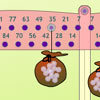Balancing 2

Age 11 to 14 Challenge Level:

Meg and Mo still need to hang their marbles so that they balance, but this time the constraints are different. Use the interactivity to experiment and find out what they need to do.The Triangle Game

Age 11 to 16 Challenge Level:

Can you discover whether this is a fair game?Rolling Around

Age 11 to 14 Challenge Level:

A circle rolls around the outside edge of a square so that its circumference always touches the edge of the square. Can you describe the locus of the centre of the circle?Instant Insanity

Age 11 to 18 Challenge Level:

Given the nets of 4 cubes with the faces coloured in 4 colours, build a tower so that on each vertical wall no colour is repeated, that is all 4 colours appear.Inside Out

Age 14 to 16 Challenge Level:

There are 27 small cubes in a 3 x 3 x 3 cube, 54 faces being visible at any one time. Is it possible to reorganise these cubes so that by dipping the large cube into a pot of paint three times you. . . .Cubic Net

Age 14 to 18 Challenge Level:

This is an interactive net of a Rubik's cube. Twists of the 3D cube become mixes of the squares on the 2D net. Have a play and see how many scrambles you can undo!Square It

Age 11 to 16 Challenge Level:

Players take it in turns to choose a dot on the grid. The winner is the first to have four dots that can be joined to form a square.Fractions and Percentages Card Game

Age 11 to 16 Challenge Level:

Match the cards of the same value.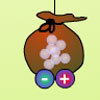Balancing 3

Age 11 to 14 Challenge Level:

Mo has left, but Meg is still experimenting. Use the interactivity to help you find out how she can alter her pouch of marbles and still keep the two pouches balanced.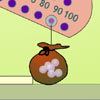Balancing 1

Age 11 to 14 Challenge Level:

Meg and Mo need to hang their marbles so that they balance. Use the interactivity to experiment and find out what they need to do.Changing Places

Age 14 to 16 Challenge Level:

Place a red counter in the top left corner of a 4x4 array, which is covered by 14 other smaller counters, leaving a gap in the bottom right hand corner (HOME). What is the smallest number of moves. . . .Drips

Age 7 to 14 Challenge Level:

An animation that helps you understand the game of Nim.Countdown Fractions

Age 11 to 16 Challenge Level:

Here is a chance to play a fractions version of the classic Countdown Game.Excel Interactive Resource: Interactive Division

Age 11 to 16 Challenge Level:

Use an Excel to investigate division. Explore the relationships between the process elements using an interactive spreadsheet.Red or Black Spinner

Age 3 to 16 Challenge Level:

A simple spinner that is equally likely to land on Red or Black. Useful if tossing a coin, dropping it, and rummaging about on the floor have lost their appeal. Needs a modern browser; if IE then at. . . .Tilting Triangles

Age 14 to 16 Challenge Level:

A right-angled isosceles triangle is rotated about the centre point of a square. What can you say about the area of the part of the square covered by the triangle as it rotates?White Box

Age 7 to 18 Challenge Level:

This game challenges you to locate hidden triangles in The White Box by firing rays and observing where the rays exit the Box.Attractive Tablecloths

Age 14 to 16 Challenge Level:

Charlie likes tablecloths that use as many colours as possible, but insists that his tablecloths have some symmetry. Can you work out how many colours he needs for different tablecloth designs?Spinners Environment

Age 5 to 18 Challenge Level:

A tool for generating random integers.Got It

Age 7 to 14 Challenge Level:

A game for two people, or play online. Given a target number, say 23, and a range of numbers to choose from, say 1-4, players take it in turns to add to the running total to hit their target.Countdown

Age 7 to 14 Challenge Level:

Here is a chance to play a version of the classic Countdown Game.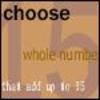Fifteen

Age 7 to 14 Challenge Level:

Can you spot the similarities between this game and other games you know? The aim is to choose 3 numbers that total 15.Interactive Number Patterns

Age 14 to 16 Challenge Level:

How good are you at finding the formula for a number pattern ?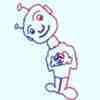Loci Resources

Age 14 to 16 Challenge Level:

This set of resources for teachers offers interactive environments to support work on loci at Key Stage 4.Proof Sorter - Quadratic Equation

Age 14 to 18 Challenge Level:

This is an interactivity in which you have to sort the steps in the completion of the square into the correct order to prove the formula for the solutions of quadratic equations.Stars

Age 11 to 14 Challenge Level:

Can you find a relationship between the number of dots on the circle and the number of steps that will ensure that all points are hit?An Unhappy End

Age 11 to 14 Challenge Level:

Two engines, at opposite ends of a single track railway line, set off towards one another just as a fly, sitting on the front of one of the engines, sets off flying along the railway line...Rollin' Rollin' Rollin'

Age 11 to 14 Challenge Level:

Two circles of equal radius touch at P. One circle is fixed whilst the other moves, rolling without slipping, all the way round. How many times does the moving coin revolve before returning to P?Excel Interactive Resource: Fraction Multiplication

Age 11 to 16 Challenge Level:

Use Excel to explore multiplication of fractions.Matter of Scale

Age 14 to 16 Challenge Level:

Prove Pythagoras' Theorem using enlargements and scale factors.